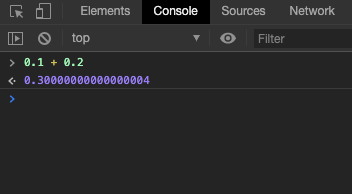#DAY 3
0
Software Development

## IEEE-754 與浮點數運算

• IEEE-754
• 規範對於工程發展的重要性
• 認識浮點數
• 使用演算法改善浮點數運算時帶來的誤差問題

## 進入正題

### IEEE-754IEEE 二進位浮點數算術標準（IEEE 754）是 20 世紀 80 年代以來最廣泛使用的浮點數運算標準，為許多 CPU 與浮點運算器所採用。這個標準定義了表示浮點數的格式（包括負零-0）與反常值（denormal number），一些特殊數值（（無窮（Inf）與非數值（NaN）），以及這些數值的「浮點數運算子」；它也指明了四種數值修約規則和五種例外狀況（包括例外發生的時機與處理方式）。
--wikipedia

### 單精度浮點數

IEEE 754 規範了四種浮點數表達方式，分別是:

• 單精確度
• 雙精確度
• 延伸單精確度
• 延伸雙精確度

• sign(1)

`1` 表示負數，反之代表正數。

• exponent(8)

8 bits 一共可表示 255 種狀態，為了有效分配指數為正數和負數的情況，IEEE-754 以 `127` 表示指數為 0 的情況，若我們今天要表示指數為 3 則變成 3 + 127 = `130`

``````    01111111
``````
• fraction(23)

## 將實際資料以浮點數表示

### 正規化

``````8.5 = 2³ + 1/2¹
2³ + 1/2¹ = 1000.1
``````

### 單精度表示

• sign
因為 8.5 是正數，所以 sign bit 存放 0。
• exponent
在剛剛，我們得到了 1.0001 x 2³ 的結果，而 exponent 就是 3。
你認為這樣就結束了嗎?
不!剛剛有提到這邊以 +127 作為 0，所以要表示 3 我們必須將 127 + 3。因此，我們在這邊會在 8 bits 中存放:
``````10000010 (130)
``````
• fraction
在處理完 sign 和 exponent 的部分後，我們只要將剩餘的小數部分處理就大功告成啦!
fraction 一共被分配到 23 個 bit，以 8.5 為例，我們僅會存放`.0001`，所以在記憶體中看起來會像是:
``````00010000000000000000000
``````

``````0 10000010 00010000000000000000000
``````

## 浮點數為什麼叫做浮點數?-- wikipedia

## 浮點數的特例以及如何應對

### 浮點數的特例• +-0
當 exponent 與 fraction 皆為 0，其值為 +-0。

• +-∞
當 exponent 為 255 且 fraction 為 0，其值為無窮大。

• NaN
當 exponent 為 255 且 fraction 不為 0，表示 Not a number。此外，根據 IEEE 754 2008 年版本可以得知 NaN 被歸類為兩種:

• Quiet NaN
• Signal NaN
``````X111 1111 1AXX XXXX XXXX XXXX XXXX XXXX
``````

我們可以透過從低位數來的第 23 個位元(也就是上面 A 所在的位置) 判斷 NaN 的種類，如果在 A 的位置為 1，則為 Quiet NaN，否則為 Signal NaN。
兩者的差別我們也可以從 Intel manual 中得知:

SNaNs are typically used to trap or invoke an exception handler. They must be inserted by software; that is, the processor never generates an SNaN as a result of a floating-point operation.

Signal NaN 不會由處理器運算產生且對其做運算會對電腦發出訊號進入異常處理。相反的，Quiet NaN 經由處理器運算後並不會造成程式終止。關於 NaN，可以在 What is the difference between quiet NaN and signaling NaN? 這個問答串中找到網友對兩種 NaN 設計的實驗:

``````#include <cassert>
#include <cfenv>
#include <cmath> // isnan
#include <iostream>
#include <limits> // std::numeric_limits
#include <unistd.h>

#pragma STDC FENV_ACCESS ON

int main() {
float snan = std::numeric_limits<float>::signaling_NaN();
float qnan = std::numeric_limits<float>::quiet_NaN();
float f;

// No exceptions.
assert(std::fetestexcept(FE_ALL_EXCEPT) == 0);

// Still no exceptions because qNaN.
f = qnan + 1.0f;
assert(std::isnan(f));
if (std::fetestexcept(FE_ALL_EXCEPT) == FE_INVALID)
std::cout << "FE_ALL_EXCEPT qnan + 1.0f" << std::endl;

// Now we can get an exception because sNaN, but signals are disabled.
f = snan + 1.0f;
assert(std::isnan(f));
if (std::fetestexcept(FE_ALL_EXCEPT) == FE_INVALID)
std::cout << "FE_ALL_EXCEPT snan + 1.0f" << std::endl;
feclearexcept(FE_ALL_EXCEPT);

// And now we enable signals and blow up with SIGFPE! >:-)
feenableexcept(FE_INVALID);
f = qnan + 1.0f;
std::cout << "feenableexcept qnan + 1.0f" << std::endl;
f = snan + 1.0f;
``````

``````g++ -ggdb3 -O0 -Wall -Wextra -pthread -std=c++11 -pedantic-errors -o blow_up.out blow_up.cpp -lm -lrt
./blow_up.out
echo \$?
``````
• 非正規數
當 exponent 為 0 且 fraction 不為 0 時，該浮點數為非正規數。用數線來看的話，大概長這樣:
``````-∞ ~ (sign = 1)最大正規數 ~ -1 ~ (sign = 1)最小正規數 ~ (sign = 1)最大的非正規數 ~ (sign = 1)最小的非正規數 ~ -0/+0 ~ (sign = 0)最小的非正規數 ~ (sign = 0)最大的非正規數 ~ (sign = 0)最小正規數 ~ (sign = 0)最大正規數  ~ +∞
``````

-- wikipedia

### Kahan Summation

#### 準確度與精密度• Accurate && Precise
3.14159
• Accurate && Inprecise
3.14
• Inaccurate && Precise
3.14128
• Inaccurate && Inprecise
3.22

#### 演算法 (虛擬碼)

``````function KahanSum(input)
var sum = 0.0                    // Prepare the accumulator.
var c = 0.0                      // A running compensation for lost low-order bits.

for i = 1 to input.length do     // The array input has elements indexed input to input[input.length].
var y = input[i] - c         // c is zero the first time around.
var t = sum + y              // Alas, sum is big, y small, so low-order digits of y are lost.
c = (t - sum) - y            // (t - sum) cancels the high-order part of y; subtracting y recovers negative (low part of y)
sum = t                      // Algebraically, c should always be zero. Beware overly-aggressive optimizing compilers!
next i                           // Next time around, the lost low part will be added to y in a fresh attempt.

return sum
``````

``````10000.0 + 3.14159 = 10003.14159
``````

``````10000.0 + 3.14159 = 10003.1
``````

Kahan Summation 的作法就是算出這些遺失的值，等到下一輪運算再將他補嘗到新的輸入當中:

``````/* 求出誤差值 */
c = (10003.1 - 10000.0) - 3.14159 //-0.04159
/* 補償 */
y = 3.00001 - (-0.04159) //假設下一輪運算的輸入是 3.00001
``````